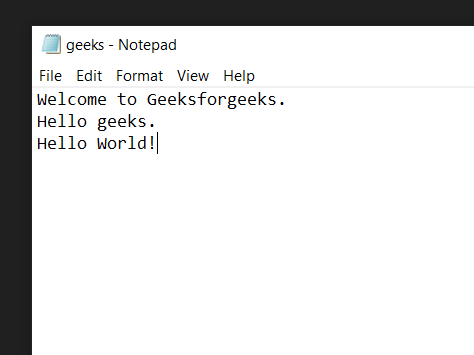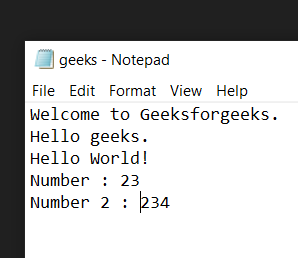# Python Program to Count Words in Text File

• Difficulty Level : Medium
• Last Updated : 14 Sep, 2021

In this article, we are going to see how to count words in Text Files using Python.

### Example 1: Count String Words

First, we create a text file of which we want to count the number of words. Let this file be SampleFile.txt with the following contents:

Attention geek! Strengthen your foundations with the Python Programming Foundation Course and learn the basics.

To begin with, your interview preparations Enhance your Data Structures concepts with the Python DS Course. And to begin with your Machine Learning Journey, join the Machine Learning - Basic Level Course

#### File for demonstration:## Python3

 `# creating variable to store the``# number of words``number_of_words ``=` `0`` ` `# Opening our text file in read only``# mode using the open() function``with ``open``(r``'SampleFile.txt'``,``'r'``) as ``file``:`` ` `    ``# Reading the content of the file``    ``# using the read() function and storing``    ``# them in a new variable``    ``data ``=` `file``.read()`` ` `    ``# Splitting the data into seperate lines``    ``# using the split() function``    ``lines ``=` `data.split()`` ` `    ``# Adding the length of the``    ``# lines in our number_of_words``    ``# variable``    ``number_of_words ``+``=` `len``(lines)`` ` ` ` `# Printing total number of words``print``(number_of_words)`

Output:

`7`

Explanation:

• Creating a new variable to store the total number of words in the text file. And then open the text file in read-only mode using the open() function.
• Read the content of the file using the read() function and storing them in a new variable. And then split the data stored in the data variable into separate lines using the split() function and then storing them in a new variable. And add the length of the lines in our number_of_words variable.

### Example 2: Count the number of words, not Integer

File for demonstration:Below is the implementation:

## Python3

 `# creating variable to store the``# number of words``number_of_words ``=` `0`` ` `# Opening our text file in read only``# mode using the open() function``with ``open``(r``'SampleFile.txt'``,``'r'``) as ``file``:`` ` `    ``# Reading the content of the file``    ``# using the read() function and storing``    ``# them in a new variable``    ``data ``=` `file``.read()`` ` `    ``# Splitting the data into seperate lines``    ``# using the split() function``    ``lines ``=` `data.split()`` ` `    ``# Iterating over every word in``    ``# lines``    ``for` `word ``in` `lines:`` ` `        ``# checking if the word is numeric or not``        ``if` `not` `word.isnumeric():          `` ` `            ``# Adding the length of the``            ``# lines in our number_of_words``            ``# variable``            ``number_of_words ``+``=` `1`` ` `# Printing total number of words``print``(number_of_words)`

Output:

`11`

Explanation: Create a new variable to store the total number of words in the text file and then open the text file in read-only mode using the open() function. Read the content of the file using the read() function and storing them in a new variable and then split the data stored in the data variable into separate lines using the split() function and then storing them in a new variable, Iterating over every word in lines using the for loop and check if the word is numeric or not using the isnumeric() function then add 1 in our number_of_words variable.

My Personal Notes arrow_drop_up